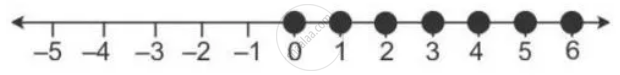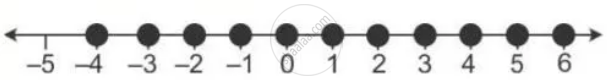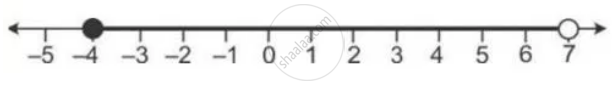Share

# Find the Range of Values of X Which Satisfy: - 1/3 <= X/2 + 1 2/3 < 5 1/6 the Graph in Each of the Following Cases the Values of X on the Different Real Number Lines: 1) X ∈ W 2) X ∈ Z 3) X ∈ R - Mathematics

Course

#### Question

Find the range of values of x which satisfy:

- 1/3 <= x/2 + 1 2/3 < 5 1/6

The graph in each of the following cases the values of x on the different real number lines:

1) x ∈ W

2) x ∈ Z

3) x ∈ R

#### Solution

-1/3 <= x/2 + 1 2/3 < 5 1/6

-1/3 - 5/3 <= x/2 < 31/6- 5/3

-6/3 <= x/2 < 21/6

-4 <= x < 7

1) if x  ∈ W, range of values of x is {0, 1, 2, 3, 4, 5, 6}2) if x ∈ Z, the range of values of x is {-4, -3, -2, -1, 0, 1, 2, 3, 4, 5, 6}3) if x ∈ R range of values of x is -4 <= x <7Is there an error in this question or solution?
Find the Range of Values of X Which Satisfy: - 1/3 <= X/2 + 1 2/3 < 5 1/6 the Graph in Each of the Following Cases the Values of X on the Different Real Number Lines: 1) X ∈ W 2) X ∈ Z 3) X ∈ R Concept: Representation of Solution on the Number Line.